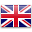Live ChatEnglish
Home / News / How To Calculate How Long A Battery Will Last

# How To Calculate How Long A Battery Will Last

Battery life is a pain point for all smartphone users. It’s also an area where manufacturers love to boast about their products. There are so many claims about battery life these days that it can be difficult to determine which one is the most reliable.

## What does battery capacity actually mean?

There are a few different factors that determine how long a battery will last. The most important one is capacity. Capacity is measured in milliamp hours (mAh) and it tells you how much charge the battery can store. If you know the capacity of a battery, you can easily calculate how long it will last. For example, if you have a battery with a 3000mAh capacity, it will last three times longer than a 1500mAh battery. Why is this important? Because you can calculate how long a battery will last. If you’re charging a smartphone, you can use the formula below to calculate how long it will take to charge.

## How to calculate the battery capacity

To calculate the capacity of a battery, you have to work out its amp hours (Ah). To do this, you’ll need to know how many Volts the battery is and how many Amps it can charge at. The best way to do this is to use a battery capacity calculator. You can find these online, or there are also some great apps that do this for you. Once you have the Ah, you can use the formula below to calculate the capacity of the battery.

## How to calculate how long a battery will last

Once you’ve worked out how much charge the battery has and how much charge it needs, you can work out how long it will last. To do this you need to know how long it will take to charge the battery. So if you have a 10,000mAh battery and it takes two hours to charge, you can work out how long it will last. You just need to use the formula below.